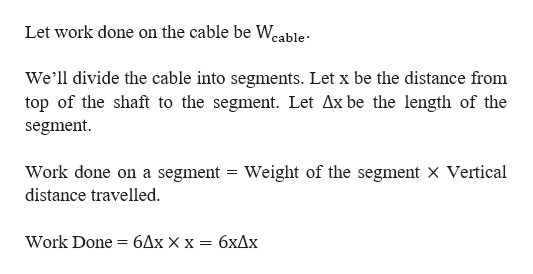# A cable that weighs 6 lb/ft is used to lift 800 lb of coal up a mine shaft 450 ft deep. Find the work done.A.) Show how to approximate the required work by a Riemann sum. (Let x be the distance in feet below the top of the shaft. Enter xi* as xi.)B.) Express the work as an integral.C.)Evaluate the integral.

Question
42 views

A cable that weighs 6 lb/ft is used to lift 800 lb of coal up a mine shaft 450 ft deep. Find the work done.

A.) Show how to approximate the required work by a Riemann sum. (Let x be the distance in feet below the top of the shaft. Enter xi* as xi.)

B.) Express the work as an integral.

C.)Evaluate the integral.

check_circle

Step 1

Given that a cable that weighs 6 lb/ft is used to lift 800 lb of coal up a mine shaft 450 ft deep

Step 2

To begin with we consider the following assumption.help_outlineImage TranscriptioncloseLet work done on the cable be Weable We'll divide the cable into segments. Let x be the distance from top of the shaft to the segment. Let Ax be the length of the segment Work done on a segment = Weight of the segment X Vertical distance travelled Work Done бДх хх — бхДх fullscreen
Step 3

b) Now, express the work...

### Want to see the full answer?

See Solution

#### Want to see this answer and more?

Solutions are written by subject experts who are available 24/7. Questions are typically answered within 1 hour.*

See Solution
*Response times may vary by subject and question.
Tagged in

### Calculus